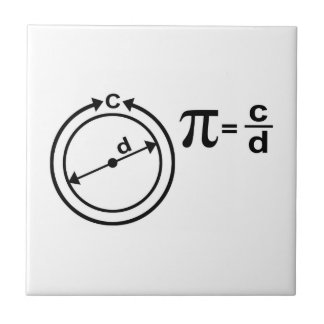# Pi

https://arbital.com/p/pi

by Michael Cohen Jun 14 2016 updated Jul 21 2016

Pi, usually written $π$, is a number equal to the ratio of a circle's [-circumference] to its [-diameter]. The value of $π$ is approximately $3.141593$.If the length of a curve seems like an ill-defined concept to you (maybe you only understand how lines could have lengths), consider bigger and bigger [-regular_polygon regular polygons] that make better and better approximations of the circle. As the number of sides $N$ of the polygon goes to $∞$, the perimeter will approach a length of $π$ times the diameter.

One could also define $π$ to be the area of a circle divided the area of a square, whose edge is the radius of the circle.

## What Kind of Number It Is

It's not an Integer.If the diameter here is 1, then the perimeter of the hexagon is 3, the perimeter of the square is 4, and the circumference of the circle is in between. There are no integers between 3 and 4.

It's not rational, nor is it algebraic. It's transcendental.